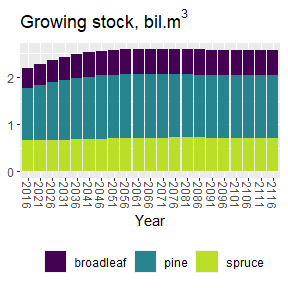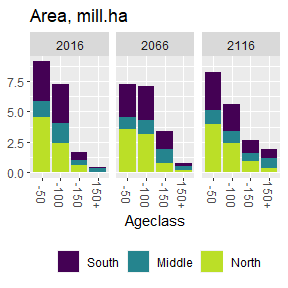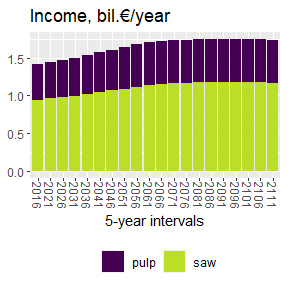# Example scenarios

library(dplyr)
library(ggplot2)
library(gridExtra)
library(efdm)
data(example)
theme_set(theme(legend.position = "bottom", axis.text.x = element_text(angle = -90)))

## Estimate transition probabilities and set up actitivies

Statespace is the collection of all possible states of classifying variables and can be obtained from actprob by removing the activity probabilities (in our case, thin = thinning, ff = final felling, noman = no management). It is used for constructing transition probabilities.

statespace <- example$actprob %>% select(-c(thin, ff, noman)) head(statespace) #> region soil sp vol age #> 1 South Mineral other 1 1 #> 2 South Mineral other 1 2 #> 3 South Mineral other 1 3 #> 4 South Mineral other 1 4 #> 5 South Mineral other 1 5 #> 6 South Mineral other 1 6 Use pair data to estimate transition probabilities for actitivies no management and thinning. head(example$noman_pairs)
#>   region    soil     sp vol0 vol1 age0 age1
#> 1  South Mineral spruce   10    7   13   14
#> 2  South Mineral spruce    8    8   20   21
#> 3  South Mineral  other    9   10    5    6
#> 4  South Mineral  other    1    1    1    2
#> 5  South Mineral  other    7    8    2    3
#> 6  South Mineral  other    7   10    5    6

Statespace and prior are used to fill the missing combinations from pairdata. Here we simply assume that age grows by one age class if no data is seen. Transitions are estimated separately for each region and species. The esimation procedure uses data from different soil types with less weight than the correct soil type.

act <- define_activity("noman", c("vol", "age"))
act <- build_statespace(act, statespace,
factors=c("soil"), by=c("region","sp"))
noman <- estimatetransprobs(act, example$noman_pairs, prior_grow("age")) act <- define_activity("thin", c("vol", "age")) act <- build_statespace(act, statespace, factors=c("soil"), by=c("region","sp")) thin <- estimatetransprobs(act, example$thin_pairs, prior_grow("age"))

Final felling sets age and volume class to the smallest class. No pairdata is needed/utilized.

ff <- define_activity("ff", c("vol", "age"))
transprobs(ff) <- unique(statespace %>% select(vol0=vol, age0=age)) %>% mutate(vol1=1, age1=1, prob=1)
#>   vol0 age0 vol1 age1 prob
#> 1    1    1    1    1    1
#> 2    1    2    1    1    1
#> 3    1    3    1    1    1
#> 4    1    4    1    1    1
#> 5    1    5    1    1    1
#> 6    1    6    1    1    1

## Example 1

The activities are collected to a list. The initial state and activity probabilities and the number of time steps are the remaining parameters of the runEFDM function

activities <- list(noman, thin, ff)
state0 <- example$initial_state actprob <- example$actprob
#>   region    soil    sp vol age noman  thin    ff
#> 1  South Mineral other   1   1 0.976 0.023 0.001
#> 2  South Mineral other   1   2 0.981 0.019 0.000
#> 3  South Mineral other   1   3 0.994 0.006 0.000
#> 4  South Mineral other   1   4 0.991 0.008 0.001
#> 5  South Mineral other   1   5 0.764 0.024 0.212
#> 6  South Mineral other   1   6 0.974 0.025 0.001

EFDM is run for 20 time steps. The length of timestep is determined by the inventory data used to be 5 years.

states1 <- runEFDM(state0, actprob, activities, 20)
#>      soil region    sp vol age        area activity time
#> 1 Mineral Middle other   1   1 101627.6564    noman    0
#> 2 Mineral Middle other   1   2  73660.4310    noman    0
#> 3 Mineral Middle other   1   3  26299.4701    noman    0
#> 4 Mineral Middle other   1   4   5982.8223    noman    0
#> 5 Mineral Middle other   1   5    379.4954    noman    0
#> 6 Mineral Middle other   1   6   1104.1126    noman    0

runEFDM produces a data.frame of areas allocated to each activity at each timestep.

To obtain total growing stock volume, volume coeffiecients are used. The volume coefficients and drain were estimated based on the species composition and changes in timber assortments linked to management activities observed in the forest inventory data.

head(example$vol_coef) #> vol sp species volume #> 1 1 other all 0.506323 #> 2 10 other all 176.677720 #> 3 11 other all 210.438071 #> 4 12 other all 245.009871 #> 5 13 other all 279.955533 #> 6 14 other all 314.900072 Drain and income…. head(example$drain_coef)
#>   vol     sp    drain assort activity
#> 1   1  other 0.000000    saw       ff
#> 2   1 spruce 0.000000    saw       ff
#> 3   2  other 0.000000    saw       ff
#> 4   2 spruce 0.000000    saw       ff
#> 5   3  other 0.000000    saw       ff
#> 6   3 spruce 1.012518    saw       ff

Income is loosely linked to the actual timber assortment prices in unit eur/m3.

head(example$income_coef) #> assort activity euro #> 1 saw ff 56 #> 2 saw thin 44 #> 3 pulp ff 20 #> 4 pulp thin 15 Simulation timesteps are mutated to mid-years of simulation steps: states1 <- states1 %>% mutate(time = factor(2016 + time*5)) Growing stock tilstate<-merge(states1, example$vol_coef) %>% mutate (volume=area*volume)
ggplot(subset(tilstate,species!='all')) + scale_fill_viridis_d(end = 0.9) +
geom_bar(aes(x=time, weight=volume/1000000000,fill=species)) +
labs(y=NULL,title=expression(paste("Growing stock, bil.",m^3)), x="Year", fill="")Age distribution

states1$ageclass <- cut(states1$age, breaks=c(0,10,20,30,35), include.lowest = TRUE,
#labels=c("0-50","51-100","101-150","150+"))
labels=c("-50","-100","-150","150+"))
states1$region <- factor(states1$region, labels = c("South", "Middle", "North"))
ggplot(subset(states1, time %in% c(2016,2066,2116))) +
geom_bar(aes(x=ageclass, weight=area/1000000, fill=region)) +
scale_fill_viridis_d(end = 0.9) +
facet_grid(cols=vars(time)) + labs(y=NULL, title="Area, mill.ha", x="Ageclass", fill=NULL)First the drain in units m3/ha is converted in into eur/ha. Then multiplication with area (ha) gives the euros.

euro<- merge(example$drain_coef, example$income_coef) %>% mutate(euro = euro*drain)
removal <- merge(states1, euro) %>% mutate(income=euro*area)

ggplot(subset(removal,!time %in% c(2116))) +
geom_bar(aes(x=time, weight=income/5000000000, fill=assort)) +
scale_fill_viridis_d(end = 0.9) +
labs(y=NULL,title = "Income, bil.€/year", x="5-year intervals", fill=NULL )## Example 2

In this example the tree species changes after final felling. Therefore we redefine final felling activity taking into account in addition to volume and age also the dominant species. The change depends on region and dominant species. Volume and age act as before. They more to the smallest classes (vol1=1 and age1=1).

ff_age_species <- define_activity("ff", c("vol", "age", "sp"))
transprobs(ff_age_species) <- unique(filter(statespace) %>%
select(vol0=vol, age0=age, sp0=sp, region)) %>%
group_by(region, sp0, vol0, age0) %>%
summarize(data.frame(vol1=1, age1=1, sp1=c('other','spruce'),
prob=case_when(sp0=='other' && region=='South' ~ c(1, 0),
sp0=='other' && region=='Middle' ~ c(1, 0),
sp0=='other' && region=='North' ~ c(0.8, 0.2),
sp0=='spruce' && region=='South' ~ c(0.3, 0.7),
sp0=='spruce' && region=='Middle' ~ c(0.2, 0.8),
sp0=='spruce' && region=='North' ~ c(0, 1))))
#> summarise() has grouped output by 'region', 'sp0', 'vol0', 'age0'. You can override using the .groups argument.
activities2 <- list(noman, thin, ff_age_species)
states2 <- runEFDM(state0, actprob, activities2, 20)

## Example 3 - Land use changes

First we add two land use classes to the statespace. Agriculture is classified according to region and soil type, while other land use is only classified accoring to region.

statespace3 <- statespace
statespace3$landuse <- "forest" agri <- unique(statespace3 %>% mutate(sp=0, vol=0, age=0, landuse="agri")) other <- unique(statespace3 %>% mutate(soil=0, sp=0, vol=0, age=0, landuse="other")) statespace3 <- rbind(statespace3, agri, other) We use separate activities for deforestation to each land use class. Variables not used by the agriculture are set to 0. Soil type and region are not changing as a result of deforestation to agriculture. defor_to_agri <- define_activity("defor_to_agri", c("vol", "age", "sp", "landuse")) transprobs(defor_to_agri) <- unique(filter(statespace3, landuse=="forest") %>% select(vol0=vol, age0=age, sp0=sp,landuse0=landuse) %>% mutate(vol1=0, age1=0, sp1=0, landuse1="agri", prob=1)) Deforestation to other land use also changes soil type to 0. defor_to_other <- define_activity("defor_to_other", c("soil", "vol", "age", "sp", "landuse")) transprobs(defor_to_other) <- unique(filter(statespace3, landuse=="forest") %>% select(soil0=soil, vol0=vol, age0=age, sp0=sp,landuse0=landuse) %>% mutate(soil1=0, vol1=0, age1=0, sp1=0, landuse1="other", prob=1)) Afforestation only applies to agriculture. Volume and age classes start from 1 and the area is split evenly to spruce and other species. affor <- define_activity("affor", c("vol", "age", "sp", "landuse")) aff <- filter(statespace3, landuse=="agri") %>% select(soil, vol0=vol, age0=age, region, sp0=sp, landuse0=landuse) %>% mutate(vol1=1, age1=1, sp1="other", landuse1="forest", prob=1) aff <- rbind(aff %>% mutate(sp1="spruce", prob=0.5), aff %>% mutate(sp1="other", prob=0.5)) transprobs(affor) <- aff A donothing activity is used for non-forest land uses, when there is nothing forestry related going on. donothing <- define_activity("donothing", character()) activities3 <- list(noman, thin, ff, defor_to_other, defor_to_agri, affor, donothing) adding land use information to state state03 <- state0 state03$landuse <- "forest"
state03 <- rbind(state03,  agri %>% mutate(area=rep(c(1552000/2,997000/2,76000/2),each=2)),
other %>% mutate(area=c(721000,507000,112000)))

Activity probabilities for new land uses

actprob3 <- actprob
actprob3$defor_to_other <- 0.0002 actprob3$defor_to_agri <- 0.00025
actprob3$affor <- 0 actprob3$landuse <- "forest"
actprob3\$donothing <- 0
actnames <- setdiff(names(actprob3), names(state03))
actprob3[actnames] <- actprob3[actnames]/rowSums(actprob3[actnames])
actprob3 <- rbind(actprob3,
agri %>% mutate(thin=0, ff=0, noman=0, defor_to_other=0, defor_to_agri=0, affor=0.0005, donothing=0.9995),
other %>% mutate(thin=0, ff=0, noman=0, defor_to_other=0, defor_to_agri=0, affor=0, donothing=1))
states3 <- runEFDM(state03, actprob3, activities3, 20)